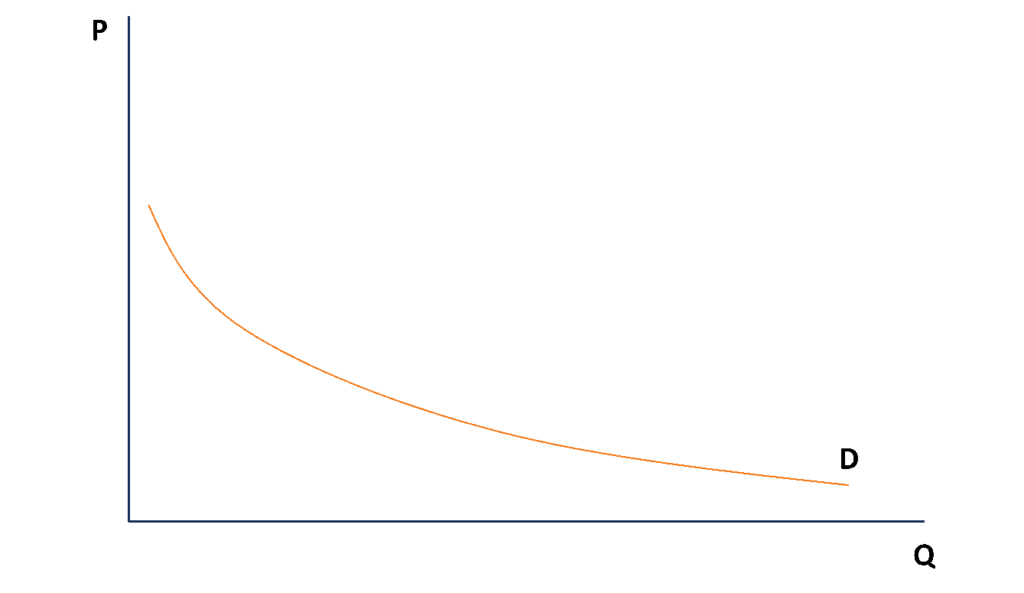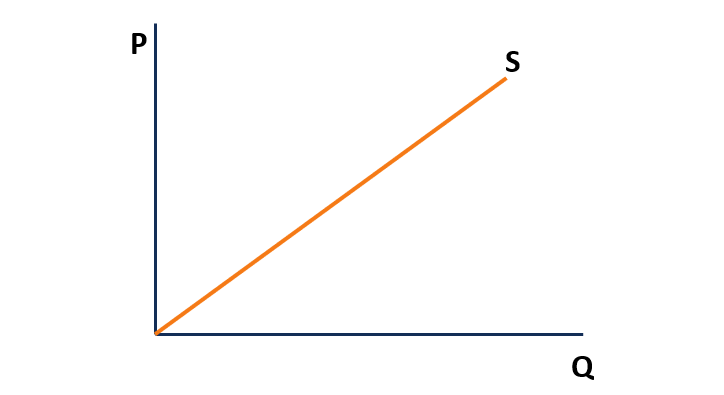# Unit Elastic

A term that describes a situation in which a change in one variable results in an equally proportional change in another variable

# What is Unit Elastic?

In economics, unit elastic (also known as unitary elastic) is a term that describes a situation in which a change in one variable results in an equally proportional change in another variable. The concept of unit elastic is primarily associated with elasticity, which is one of the fundamental concepts in economics. In this context, elasticity indicates the sensitivity of one variable in response to the changes in another variable.Economists use elasticity primarily to assess the demand or supply of a good in response to changes in the price of a good or income of consumers. As such, the term “unit elasticity” is frequently used to describe demand or supply curves that are perfectly responsive to price changes.

Note that it is extremely difficult to encounter unit elastic goods.  In most cases, a good is either elastic or inelastic relative to market changes.

### Unit Elastic Demand

Unit elastic demand is referred to as a demand in which any change in the price of a good leads to an equally proportional change in quantity demanded. In other words, the unit elastic demand implies that the percentage change in quantity demanded is exactly the same as the percentage change in price. Demand elasticity of a good with unit elastic demand is 1 (strictly speaking, elasticity equals -1 since the demand curve is downward sloping; but in most cases, elasticity is calculated as an absolute value).

The concept of elasticity comes with some crucial implications for businesses. If a company sells goods with unit elastic demand, it must carefully assess its pricing strategy. The main reason is that a substantial change in price will result in a substantial change in the quantity demanded.

Obviously, significant changes in demand can significantly impact a company’s profitability. For example, if it sells smartphones with unit elastic demand, a 10% price increase will lead to a 10% decrease in the quantity demanded. Thus, the company’s revenue will decline by 10% as well.

Graphically, unit elastic demand is depicted as a curve rather than a straight line.### Unit Elastic Supply

Unit elastic supply is referred to as a supply that is perfectly responsive to price changes. In other words, any change in the price of a good with unit elastic supply results in an equally proportional change in quantity supplied. Supply elasticity of a good with unit elastic supply is 1 (unlike the demand curve, the supply curve is upward sloping; thus, the elasticity of unit elastic supply is simply 1).

Similar to unit elasticity of demand, unit elasticity of supply has great implications in a business context. For example, if a company produces goods with unit elastic supply, it indicates that the company’s production capacities should take into consideration price fluctuations. If the price of a good changes significantly, a company should respond with a respective change in its production.

Graphically, unit elastic supply is depicted as a straight line that starts from the origin (point 0;0).